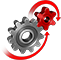# WolframSystemModeler

## Piston Engine

In a piston engine, the flywheel stores rotational energy that enables the piston to return to its original position. In this example model of a piston engine, we will use Mathematica animations to show how the rotational energy is continuously stored and used.

### The Piston Engine ModelThe piston engine is modeled in the Planar Mechanics library using only revolute and prismatic joints, making it a completely planar model. The flywheel has an initial angular velocity of -2.5 rad/s in order to demonstrate the movement of the engine.
The bottom‐left animation shows the system from a two‐dimensional point of view. The bottom‐right animation shows the same system, but in three dimensions. In other words, we have taken a completely planar model and used Mathematica graphics to illustrate it in a higher dimension. The top plot illustrates how the stored rotational energy changes as the piston moves.

Build 2D mechanical models with the Planar Mechanics library

The two‐dimensional piston engine model can be animated in both 2D and 3D.

## Wolfram System Modeler

Questions? Comments? Contact a Wolfram expert »